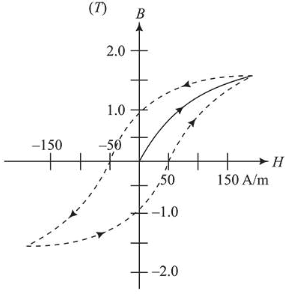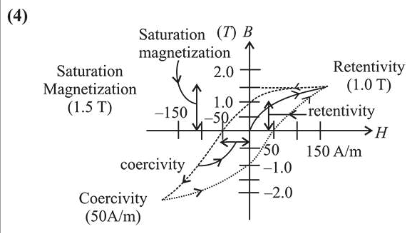# The figure gives experimentally measured B vs.Question:The figure gives experimentally measured $B$ vs. $H$ variation in a ferromagnetic material. The retentivity, co-ercivity and saturation, respectively, of the material are:

1. (1) $1.5 \mathrm{~T}, 50 \mathrm{~A} / \mathrm{m}$ and $1.0 \mathrm{~T}$

2. (2) $1.5 \mathrm{~T}, 50 \mathrm{~A} / \mathrm{m}$ and $1.0 \mathrm{~T}$

3. (3) $150 \mathrm{~A} / \mathrm{m}, 1.0 \mathrm{~T}$ and $1.5 \mathrm{~T}$

4. (4) $1.0 \mathrm{~T}, 50 \mathrm{~A} / \mathrm{m}$ and $1.5 \mathrm{~T}$

Correct Option: 2,

Solution: